## Chemical Reaction with Autocatalytic Step

Original source of the model: H.-J. Krug, L. Kuhnert: Ein oszillierendes Modellsystem mit autokatalytischem Teilschritt. Z. Phys. Chemie 266 (1985) 65-73.

The flow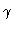describes the input of y1; y1,y2,y3 are dimensionless variables. For large values of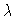, a relaxation oscillation develops.

Figure 1
Stable solutions,for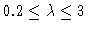; the time is normalized to unity. Stationary and periodic orbits meet in a Hopf bifurcation.

Figure 2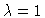, projection to the (y1,y2) plane. Initial values: y1=2, y2=y3=1. Dynamics of a stable focus.

Figure 3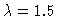, projection to the (y1,y2) plane. Initial values: y1=2, y2=y3=1. Dynamics of a stable limit cycle.

Figure 4
Bifurcation diagram y1(0) versus; Hopf bifurcation at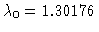.

This Example
Figure 1
Figure 2
Figure 3
Figure 4